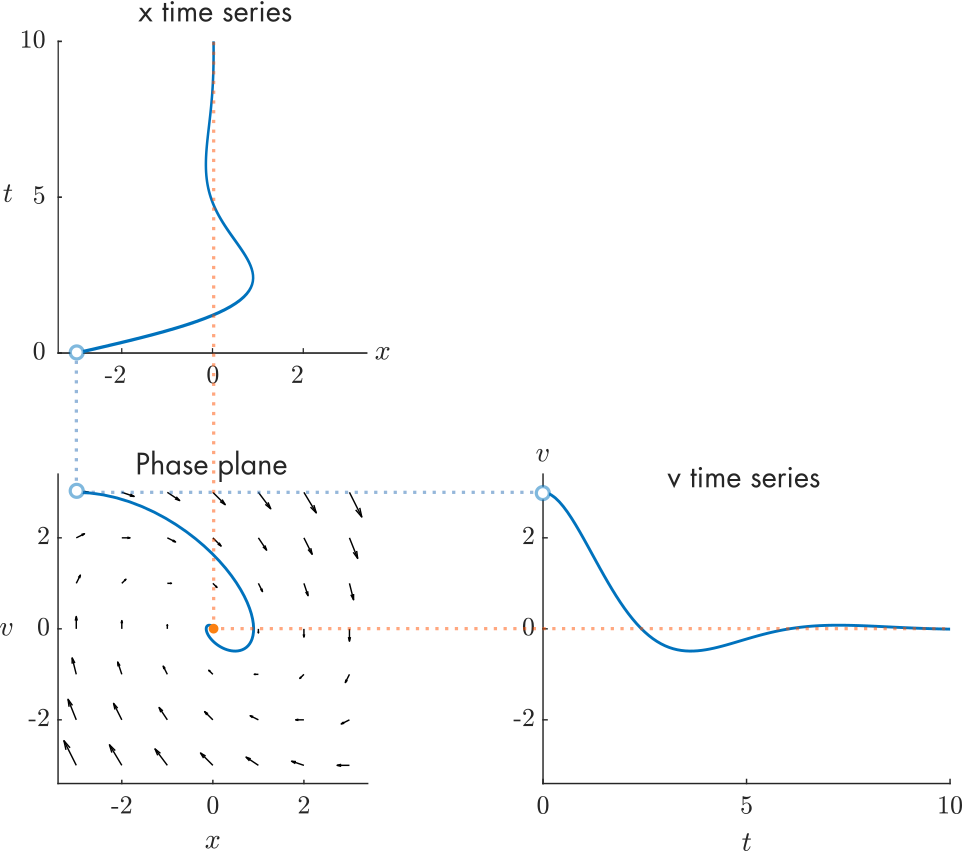## Qualitative Analysis of ODEs

versión 1.1.0 (3.03 MB) por
Interactive courseware module that addresses the qualitative analysis of ordinary differential equations taught in mathematics courses.

315 descargas

Actualizada 27 Dec 2021

De GitHub

# Qualitative Analysis of ODEsCurriculum Module
Created with R2021a. Compatible with R2021a and later releases.## Description

This curriculum module teaches qualitative analysis of ordinary differential equations (ODEs) using interactive live scripts and MATLAB® apps. The first two scripts consider first order ODEs. In these scripts, students draw solutions on a slope field, identify and classify equilibria, and draw phase line portraits. In the second half of the module, students analyze systems of two autonomous ODEs. In this part, students create phase plane portraits, solve for equilibria, and classify equilibria of linear systems by examining the eigenvalues of the coefficient matrix. Throughout the module, students apply the mathematical tools to study an energy balance climate model and a mass-spring-damper model.

The instructions inside the live scripts will guide you through the exercises and activities. Get started with each live script by running it one section at a time. To stop running the script or a section midway (for example, when an animation is in progress), use the Stop button in the RUN section of the Live Editor tab in the MATLAB Toolstrip.

## Details

`Part1_SlopeFields.mlx, Part1_SlopeFieldsSoln.mlx`
An interactive lesson that teaches how to analyze first order ODEs using slope fields.

Learning Goals:

• Classify ODEs as autonomous or non-autonomous
• Describe how a slope field is generated
• Draw ODE solutions through the slope field by hand
• Explain the physical meaning of terms in an energy balance climate model
• Plot solutions of the energy balance climate model

`Part2_Equilibria1D.mlx, Part2_Equilibria1DSoln.mlx`
A live script lesson that explains how to find and classify equilibria of first order, autonomous differential equations.

Learning Goals:

• Solve for equilibria of autonomous first order ODEs
• Classify equilibria as stable, unstable, or semi-stable
• Draw phase line portraits of autonomous ODEs and relate them to the slope field
• Find the equilibria of the energy balance climate model
• Describe the real-world meaning of the climate model equilibria

`Part3_PhasePlanes.mlx, Part3_PhasePlanesSoln.mlx`
In this lesson, students learn to write a second order ODE as a system of two first order ODEs and analyze the system using the phase plane.

Learning Goals:

• Write a second order ODE as a first order ODE system
• Describe how a phase plane is generated
• Relate solutions plotted on the phase plane to time series solution plots
• Compare behaviors of the mass-spring-damper model under variations in the damping coefficient

`Part4_LinearPhasePlaneAnalysis.mlx, Part4_LinearPhasePlaneAnalysisSoln.mlx`
An interactive lesson that explains how to find and classify equilibria of two dimensional, linear ODE systems.

Learning Goals:

• Find equilibria of a second order ODE system
• Compute the eigenvalues of the coefficient matrix of a linear ODE system
• Classify equilibria as nodes, saddles, spirals, or centers
• Categorize the behavior of the mass-spring-damper model by analyzing its coefficient matrix

## Products

MATLAB, Symbolic Math Toolbox™

The license for this module is available in the LICENSE.md file in this GitHub repository.

## Educator Resources

Have any questions or feedback? Contact the MathWorks online teaching team.

Copyright 2021 The MathWorks, Inc.

### Citar como

Brian Hong (2022). Qualitative Analysis of ODEs (https://github.com/MathWorks-Teaching-Resources/Qualitative-Analysis-of-ODEs/releases/tag/v1.1.0), GitHub. Recuperado .

##### Compatibilidad con la versión de MATLAB
Se creó con R2021a
Compatible con la versión R2021a y siguientes
##### Compatibilidad con las plataformas
Windows macOS Linux

### Community Treasure Hunt

Find the treasures in MATLAB Central and discover how the community can help you!

Start Hunting!
Para consultar o informar de algún problema sobre este complemento de GitHub, visite el repositorio de GitHub.
Para consultar o informar de algún problema sobre este complemento de GitHub, visite el repositorio de GitHub.